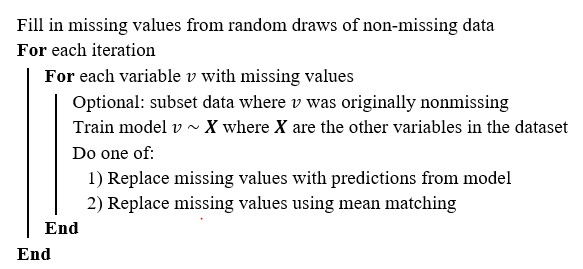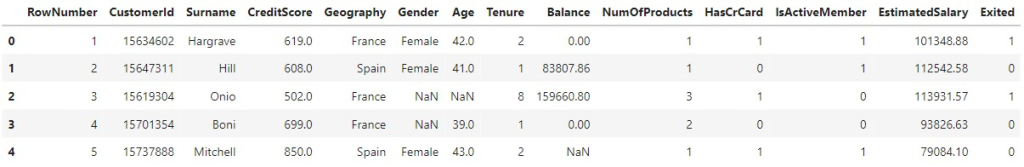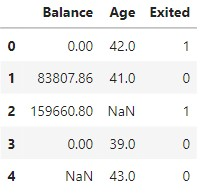# MICE imputation – How to predict missing values using machine learning in Python

MICE Imputation, short for ‘Multiple Imputation by Chained Equation’ is an advanced missing data imputation technique that uses multiple iterations of Machine Learning model training to predict the missing values using known values from other features in the data as predictors.

## What is MICE Imputation?

You can impute missing values by predicting them using other features from the dataset.

The MICE or ‘Multiple Imputations by Chained Equations’, aka, ‘Fully Conditional Specification’ is a popular approach to do this. This is quite popular in the R programming language with the mice package.

It is currently under experimental implementation in Python via sklearn package’s IterativeImputer. fancyimpute is another nice package that implements this.

## How does MICE algorithm work?

Here is a quick intuition (not the exact algorithm)

1. You basically take the variable that contains missing values as a response ‘Y’ and other variables as predictors ‘X’.

2. Build a model with rows where Y is not missing.

3. Then predict the missing observations.

Do this multiple times by doing random draws of the data and taking the mean of the predictions.Above was short intuition about how the MICE algorithm roughly works.

However, the full algorithm is more rigorous and works as follows. The following work by Azur et al talks about it in detail.

## The MICE Algorithm (Step-by-step)

For simplicity, let’s assume the dataset contains only 3 columns: A, B, C each of which contains missing values spread randomly.

The following steps are performed to perform MICE imputation:

1. Decide on the number of iterations (k) and create as many copies of the raw dataset.

2. In each column, replace the missing values with an approximate value like the ‘mean’, based on the non-missing values in that column. This is a temporary replacement. At the end of this step, there should be no missing values.

3. For the specific column you want to impute, eg: columm A alone, change the imputed value back to missing.

4. Now, build a regression model to predict A using (B and C) as predictors. For this model, only the non-missing rows of A are included. So, A is the response, while, B and C are predictors. Use this model to predict the missing values in A.

5. Repeat steps 2-4 for columns B and C as well.

Completing 1 round of predictions for columns A, B and C forms 1 iteration.

Do this for the ‘k’ iterations you have predecided. _With each iteration the predicted value of the temporary prediction for each column will keep improving._ So, there is a continuity between the successive iteration, hence the name ‘chained’.

By the end of the ‘kth iteration, the latest prediction (imputation) for each of the variables will be retained as the final imputation.

## Implement MICE with IterativeImputer from sklearn

Import the IterativeImputer and enable it.

# need to enable iterative imputer explicitly since its still experimental
from sklearn.experimental import enable_iterative_imputer
from sklearn.impute import IterativeImputer

Initialize the IterativeImputer.

The default value for the number of iterations is specified using the max_iter argument and is taken as 10. You might want to increase this if there are many missing values and takes more iterations to be accurate.

# Define imputer
imputer = IterativeImputer(random_state=100, max_iter=10)
 

Import and View dataset

import pandas as pd
file_path = "https://raw.githubusercontent.com/selva86/datasets/master/Churn_Modelling_m.csv"
df.head()Let's take only the numeric features for training. This is not a required step though.

# Use Numeric Features
df_train = df.loc[:, ["Balance", "Age", "Exited"]]
df_train.head()Train the imputer model.

# fit on the dataset
imputer.fit(df_train)
 

Predict the missing values. This is done using the transform method.

df_imputed = imputer.transform(df_train)
df_imputed[:10]
 

Output:

array([[0.00000000e+00, 4.20000000e+01, 1.00000000e+00],
[8.38078600e+04, 4.10000000e+01, 0.00000000e+00],
[1.59660800e+05, 4.47681408e+01, 1.00000000e+00],
[0.00000000e+00, 3.90000000e+01, 0.00000000e+00],
[7.25930035e+04, 4.30000000e+01, 0.00000000e+00],
[1.13755780e+05, 4.40000000e+01, 1.00000000e+00],
[0.00000000e+00, 5.00000000e+01, 0.00000000e+00],
[1.15046740e+05, 2.90000000e+01, 1.00000000e+00],
[1.42051070e+05, 4.40000000e+01, 0.00000000e+00],
[1.34603880e+05, 2.70000000e+01, 0.00000000e+00]])

Replace the imputed values.

# Replace with imputed values
df.loc[:, ["Balance", "Age", "Exited"]] = df_imputed
df.head(10)The missing values are all imputed. That's it.

You can also do MICE imputation with LightGBM based approach using a more recent library - miceforest.

## MICE Imputation with LightGBM using miceforest

MICE imputation can be made more efficient using the miceforest package. It is expected to perform significantly better because it implements lightgbm algorithm in the backend to do the imputation.

LightGBM is known for its high accuracy of predictions. Combining that power with the mice algorithm makes it a strong algorithm for imputations.

Here are some more compelling advantages:

1. It can handle categorical variables for imputations.
2. You can customize how imputation happens.
3. It's very fast. Can use GPU to go even faster.
4. Data can be imputed in place to save memory.

Let's first install the miceforest dataset.

!pip install miceforest --no-cache-dir
 

To get the latest development version, it's available in the github repository. Use the following command to install it.

!pip install git+https://github.com/AnotherSamWilson/miceforest.git
 

Import miceforest

import miceforest as mf
 

We have the original data with missing values in df_train. Let's try to impute the missing values in the data with miceforest.

# Create kernel.
kds = mf.ImputationKernel(
df_train,
save_all_iterations=True,
random_state=100
)

# Run the MICE algorithm for 2 iterations
kds.mice(2)

# Return the completed dataset.
df_imputed = kds.complete_data()
 

View original data with missing values.

df_train.head()
 

Balance Age Exited
0 0.00 42.0 1
1 83807.86 41.0 0
2 159660.80 NaN 1
3 0.00 39.0 0
4 NaN 43.0 0

View Imputed dataset

df_imputed.head()
 

Balance Age Exited
0 0.00 42.0 1
1 83807.86 41.0 0
2 159660.80 55.0 1
3 0.00 39.0 0
4 133214.88 43.0 0

It has predicted a value of '55' for the missing record.

Let's run for 5 more iterations and predict again.

kds.mice(iterations=5, n_estimators=50)
 

Now, let's predict.

df_imputed2 = kds.complete_data()
df_imputed2.head()
 

Balance Age Exited
0 0.00 42.0 1
1 83807.86 41.0 0
2 159660.80 39.0 1
3 0.00 39.0 0
4 178074.33 43.0 0

The prediction has now changed from 55 to 39.

Let's compare the actual value by loading the original data that does not contain the missing values.

import pandas as pd
file_path = "https://raw.githubusercontent.com/selva86/datasets/master/Churn_Modelling.csv"
dfs[['Balance', 'Age', 'Exited']].head()
 

Balance Age Exited
0 0.00 42 1
1 83807.86 41 0
2 159660.80 42 1
3 0.00 39 0
4 125510.82 43 0

The actual value of the data is 42, so the result gor more closer to the actual after running for 5 iterations.

This is not a conclusive check. It will be a better idea to check the imputed value for all the missing observations in the data.

So, that's how you can use miceforest.

## Similar Articles

### Logistic Regression – A Complete Tutorial With Examples in RCourse Preview

## Machine Learning A-Z™: Hands-On Python & R In Data Science

### Free Sample Videos:#### Machine Learning A-Z™: Hands-On Python & R In Data Science#### Machine Learning A-Z™: Hands-On Python & R In Data Science#### Machine Learning A-Z™: Hands-On Python & R In Data Science#### Machine Learning A-Z™: Hands-On Python & R In Data Science